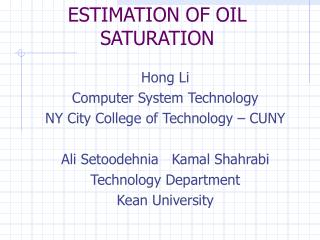DownloadDownload PresentationESTIMATION OF OIL SATURATION

# ESTIMATION OF OIL SATURATION

Télécharger la présentation## ESTIMATION OF OIL SATURATION

- - - - - - - - - - - - - - - - - - - - - - - - - - - E N D - - - - - - - - - - - - - - - - - - - - - - - - - - -
##### Presentation Transcript

1. ESTIMATION OF OIL SATURATION Hong Li Computer System Technology NY City College of Technology – CUNY Ali Setoodehnia Kamal Shahrabi Technology Department Kean University

2. introduction • Estimation of oil saturation has been an important issue for petroleum engineer • Collectable data includes pressure, rock type, depth and etc. • Permeability and saturation are not easy to measure during their study of the oil fields • Engineers attempt to determine parameters that produce the best match with observation.

3. Using Leverett J function to estimate initial oil saturation has become the problem of parameter estimation by applying different fitting functions J value Fitting function saturation pressure Leverett J function

4. Fitting fuctions • Benson-Anli • Brooks-Coery • Thomeer • O'Meara Unimodel • O’Meara Bimodel

5. Assumptions • Suppose that saturation S is function of Leverett J function with unknown parameters a = ( a1, a2, …, an), i.e. S = S(J, a), where J function value is determined by capillary pressure. • (Ji, Smi ) is a set of measured data, J function value and saturation

6. Problem statement • Determine parameters (ak) in fitting functions that produce the best match with observation, in the sense that minimizes an objective function depended on parameters (ak). • objective function is defined as

7. Numerical method • A numerical method of optimization generally consists of three steps: • Choose a starting point, i.e. given initial value of parameters. • Designate a way to generate a search sequence, A1,… An, such that E(Ak) < E(Ak-1) 3. Stipulate a convergence criterion

8. Search algorithm • The search sequence has the following general form: Ak = Ak +λk Dk • Search method: it only utilizes values of objective function • Gradient method: It utilizes gradients of objective function. Gradient method takes negative gradient direction as search direction. Dk = -E(Ak)

9. Newton Method • Newton Method: It utilizes the gradient of objection function and Hessian matrix (second order derivatives of objection function with respect to parameters), denoted by G and set the search direction Dk = -G-1E(Ak)

10. Advantage and disadvantage • rapidly converge and be more robust when number of parameters is small • When is not close to the minimum, is not necessarily positive definite

11. Given initial guess of parameters, , suppose that the first derivative of E() with respect to parameters is denoted by E() and the second derivative of E() with respect to parameters is called Hessian matrix, denoted by • G= 2 E() / i j

12. Modified Newton Method • A descent algorithm using the Newton (or near Newton) direction. • E() = E(0) +(-  0 )E(-  0 ) • + (-  0 )G (-  0 ) • so, E() = E(0) +G (-  0 ) • Set E()=0 to determined the next iteration point •  =  0 +G-1 E(0)

13. For the Newton direction to be a descent direction, we must have that the Hessian matrix G be positive definite •  chosen to assure that G+I is invertible and satisfies

14. Summary • The modified Newton method • applied the second order derivatives of the objective function with respect to the parameters • promised convergence in computer simulation. • Numerical analysis was driven to prove the problem solvability and the convergence. • Computer simulation with collected data from oil field has shown improvement in convergence speed and estimation accuracy.

15. Future Research • Neural Network has been widely applied in different fields to solve problem with parameter estimation • Preliminary research was done to estimate the oils saturation in simplified situation. • Prospect of neural network applied in saturation estimation